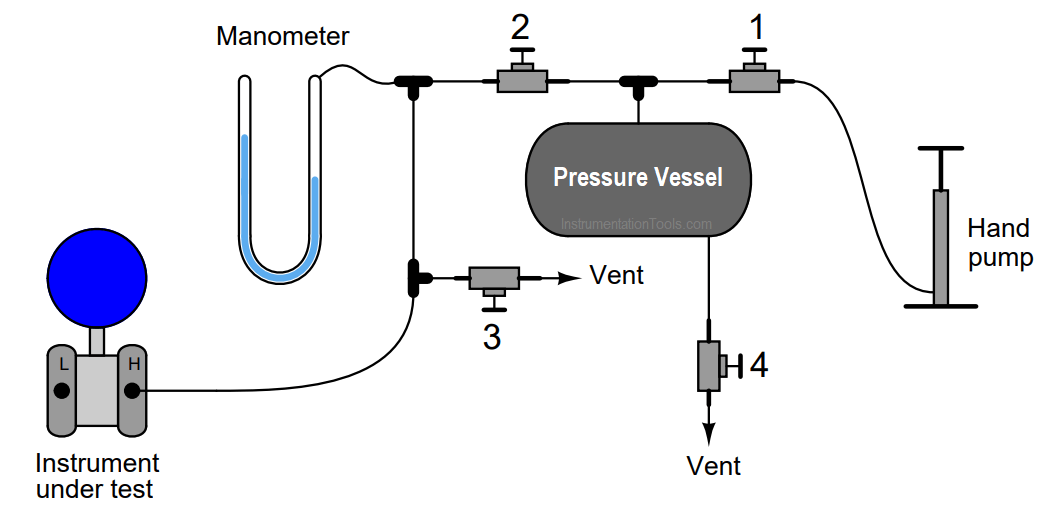# Why Pressure Vessel Connected to Tubing System?

A very useful principle in physics is the Ideal Gas Law, so called because it relates pressure, volume, molecular quantity, and temperature of an ideal gas together in one neat mathematical expression:

#### PV = nRT

Where,

P = Absolute pressure (atmospheres)

V = Volume (liters)

n = Gas quantity (moles)

R = Universal gas constant (0.0821 L · atm / mol · K)

T = Absolute temperature (K)

Although this “law” is not perfectly accurate for real gases, especially at high pressures and/or near the point of liquefaction, it is quite accurate for air near ambient temperature and pressure.

One very practical application of this law is found in a method for generating low air pressures such as those easily measured by water- or oil-based manometers.

Most mechanical air compressors generate pressures far exceeding the range of all but the largest manometers.

Though it is possible to purchase precision pressure regulators for reducing such large pressures down to a level measurable by a manometer, these devices are expensive. An alternative is to generate the air pressure with a hand pump (such as a bicycle tire pump) connected to a relatively large pressure vessel:Without the volume of the pressure vessel connected to the tubing system, the air pressure would increase dramatically for each stroke of the air pump. With the pressure vessel connected, each pump stroke contributes a much smaller amount of additional pressure to the system. Use the Ideal Gas Law equation to explain why this is.

Actuating the hand pump introduces more air molecules to the system (n).

Assuming temperature (T) remains constant, the air pressure (P) will increase in inverse proportion to the volume (V ) of the pressure vessel for each additional stroke of the pump.

#### More Questions for You:

Follow-up question: If we wished the pressure to increase less for every stroke of the pump, would we want a smaller pressure vessel or a larger pressure vessel? Explain your answer.

Challenge question: suppose a technician follows these steps in using this system.

• Close valve 2, open valves 1 and 3
• Pump several strokes’ worth of air into the pressure vessel
• Close valves 1 and 3
• Slowly open valve 2 until manometer registers desired pressure, then close

Is the air pressure going to the instrument under test greater than, less than, or equal to the air pressure in the vessel?